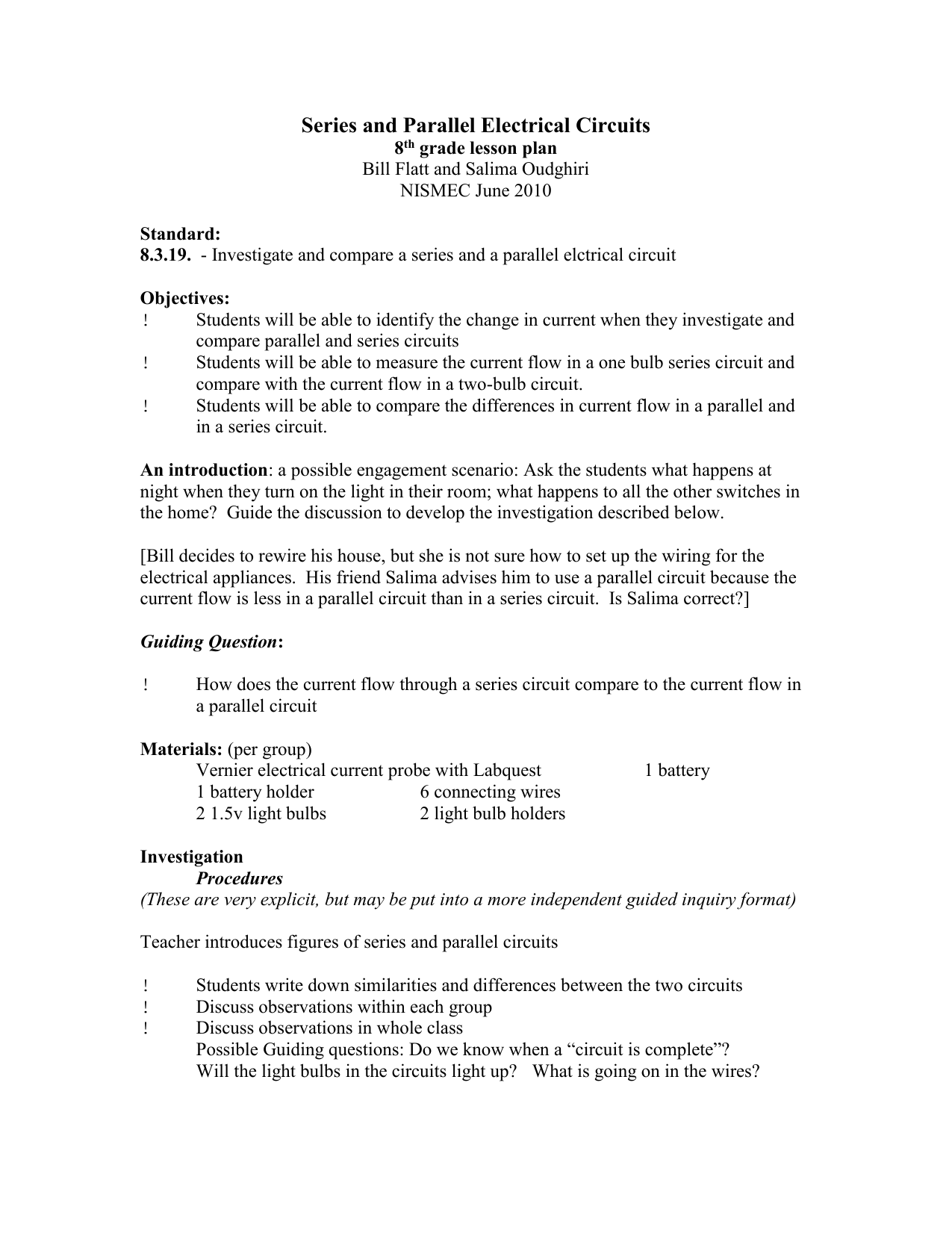# Series And Parallel Circuits Grade 8

By | August 17, 2023

## Series and Parallel Circuits

In this article, we will learn about series and parallel circuits. We will discuss the differences between these two types of circuits, and we will learn how to calculate the total resistance, current, and voltage in each type of circuit. ## Series Circuits In a series circuit, the components are connected in a single loop. This means that the current flows through each component in the circuit in the same direction. The total resistance of a series circuit is equal to the sum of the individual resistances of the components in the circuit. This can be expressed mathematically as follows: ``` Rtotal = R1 + R2 + R3 + ... + Rn ``` where: * Rtotal is the total resistance of the circuit * R1, R2, R3, ..., Rn are the individual resistances of the components in the circuit The current in a series circuit is the same at all points in the circuit. This is because the current cannot flow through any other path except through the components in the circuit. The voltage across each component in a series circuit is different. This is because the voltage drop across each component is equal to the current flowing through the component multiplied by the resistance of the component. ## Parallel Circuits In a parallel circuit, the components are connected in separate branches. This means that the current can flow through any of the branches in the circuit. The total resistance of a parallel circuit is less than the resistance of any of the individual components in the circuit. This can be expressed mathematically as follows: ``` 1 / Rtotal = 1 / R1 + 1 / R2 + 1 / R3 + ... + 1 / Rn ``` where: * Rtotal is the total resistance of the circuit * R1, R2, R3, ..., Rn are the individual resistances of the components in the circuit The current in a parallel circuit is divided between the branches in the circuit. The amount of current flowing through each branch is inversely proportional to the resistance of the branch. The voltage across each component in a parallel circuit is the same. This is because the voltage drop across each component is equal to the total current flowing through the circuit multiplied by the resistance of the component. ## Comparing Series and Parallel Circuits The following table compares series and parallel circuits: | Feature | Series Circuit | Parallel Circuit | |---|---|---| | Components connected | In a single loop | In separate branches | | Total resistance | Rtotal = R1 + R2 + R3 + ... + Rn | 1 / Rtotal = 1 / R1 + 1 / R2 + 1 / R3 + ... + 1 / Rn | | Current | Same at all points in the circuit | Divided between the branches | | Voltage | Different across each component | Same across each component | ## Applications of Series and Parallel Circuits Series and parallel circuits are used in a variety of applications. Some common applications include: *

### Electrical wiring:

Series circuits are often used in electrical wiring to connect light bulbs in a string. This ensures that all of the light bulbs will turn on when the switch is flipped. *

### Speakers:

Parallel circuits are often used in speakers to connect the speakers to the amplifier. This ensures that each speaker receives the same amount of power. *

### Computers:

Series and parallel circuits are used in computers to connect the different components of the computer. This allows the components to communicate with each other. ## Conclusion Series and parallel circuits are two important types of circuits that are used in a variety of applications. In this article, we learned about the differences between these two types of circuits, and we learned how to calculate the total resistance, current, and voltage in each type of circuit.Series Parallel Dc Circuits Worksheet ElectricSeries And Parallel Circuit 2 Worksheet18 1 Series Circuits And Parallel SiyavulaSeries And Parallel Circuits WorksheetElectric Circuits Worksheets And Online ExercisesTypes Of Circuits Parallel Circuit Series Properties VariancesHow To Solve Parallel Circuits 10 Steps With Pictures Wikihow18 1 Series Circuits And Parallel SiyavulaCircuit Diagram And Its Components Explanation With SymbolsHow Series And Parallel Circuits Are Diffe A Plus Topper18 1 Series Circuits And Parallel SiyavulaSeries And Parallel Circuits WorksheetSeries And Parallel Circuits Science Project Education Com8th Seriesandparallelcircuits1